# 如何用序列分类方式进行法律要素与当事人关联性分析

2020/10/31 18:37

2020“睿聚杯”全国高校法律科技创新大赛，是面向全国高校开展的一场高水平的法律科创竞赛。本文介绍了比赛冠军团队采用的技术方案，该方案的优势在于其基于百度飞桨平台实现，使用ERNIE作为预训练模型，并以“序列分类”为主要思路完成比赛项目方案。该方案最终以F1=91.991的成绩取得了第一名，相比Baseline的分数提高了3.267。

下载安装命令

## CPU版本安装命令

## GPU版本安装命令
pip install -f https://paddlepaddle.org.cn/pip/oschina/gpu paddlepaddle-gpu

### 数据样例

• 文 号：（2016）豫1402刑初53号

• 段落内容：商丘市梁园区人民检察院指控：1、2015年7月17日、18日，被告人刘磊、杜严二人分别在山东省单县中心医院和商丘市工行新苑盗窃现代瑞纳轿车两辆，共价值人民币107594元。其中将一辆轿车低价卖给被告人苗某某，被告人苗某某明知是赃车而予以收购。公诉机关向法庭提供了被告，是被告人供述、被害人陈述、证人证言、鉴定意见、有关书证等证据，认为被告刘磊、杜严的行为触犯了《中华人民共和国刑法》第二百六十四条之规定，构成盗窃罪。系共同犯罪。被害人苗某某的行为触犯了《中华人民共和国刑法》第二百一十二条第一款之规定，构成掩饰、隐瞒犯罪所得罪。请求依法判处。

• 被告人集合：[“刘磊”，“杜严”，“苗某某”]

• 句 子：1、2015年7月17日、18日，被告人刘磊、杜严二人分别在山东省单县中心医院和商丘市工行新苑盗窃现代瑞纳轿车两辆，共价值人民币107594元

• 要素原始值：盗窃现代瑞纳轿车

• 要素名称：盗窃、抢劫、诈骗、抢夺的机动车

• 被告人：[“刘磊”]

Baseline(official)

##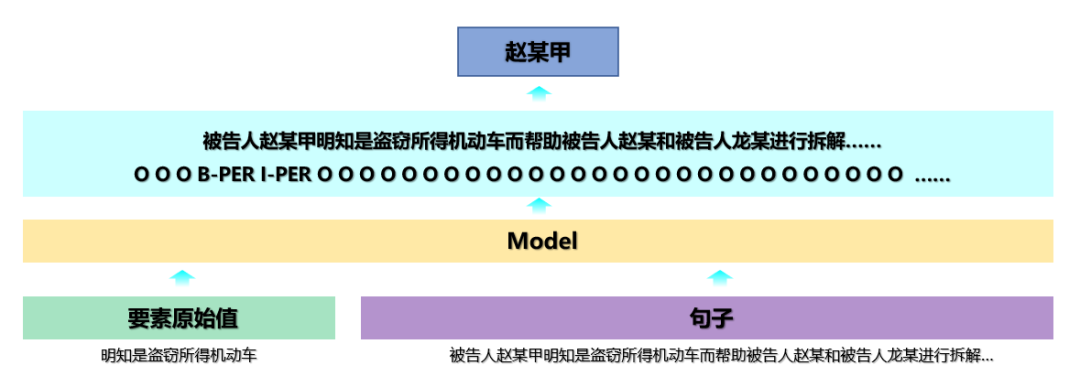Baseline方案采用的NER形式对于句子中没有人名的情况和包含多个人名的情况效果较差，因此我们结合给定的数据重新构思赛题方案。考虑到数据中已经给定了被告人集合，我们将赛题任务重新定义为序列分类任务，如图2所示。将被告人、要素名称以及句子作为输入，判断输入的被告人是否与给定要素名称相关，若相关则模型预测1，否则预测0。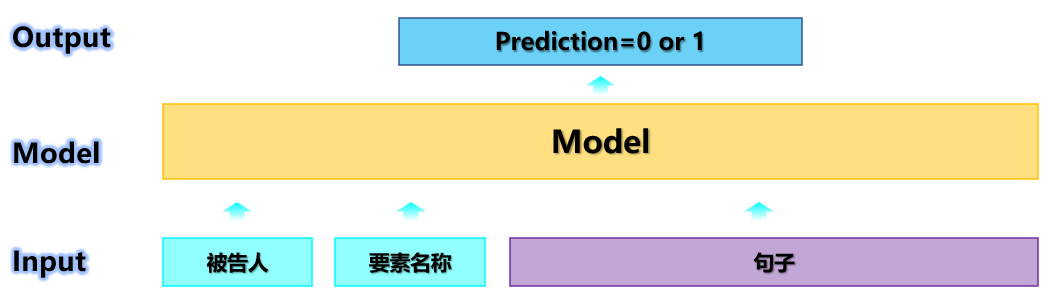### 模型描述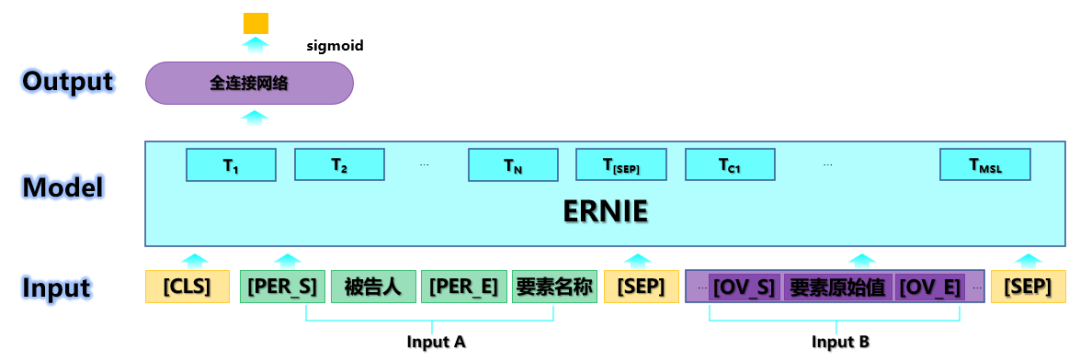Model核心代码：

class ErnieForElementClassification(ErnieModel):
def __init__(self, cfg, name=None):
super(ErnieForElementClassification, self).__init__(cfg, name=name)
initializer = F.initializer.TruncatedNormal(scale=cfg['initializer_range'])
self.classifier = _build_linear(cfg['hidden_size'], cfg['num_labels'], append_name(name, 'cls'), initializer)
prob = cfg.get('classifier_dropout_prob', cfg['hidden_dropout_prob'])
self.dropout = lambda i: L.dropout(i, dropout_prob=prob,   dropout_implementation="upscale_in_train",) if self.training else i

def forward(self, *args, **kwargs):
labels = kwargs.pop('labels', None)
pooled, encoded = super(ErnieForElementClassification, self).forward(*args, **kwargs)
hidden = self.dropout(pooled)
logits = self.classifier(hidden)
logits = L.sigmoid(logits)
sqz_logits = L.squeeze(logits, axes=)
if labels is not None:
if len(labels.shape) == 1:
labels = L.reshape(labels, [-1, 1])
part1 = L.elementwise_mul(labels, L.log(logits))
part2 = L.elementwise_mul(1-labels, L.log(1-logits))
loss = - L.elementwise_add(part1, part2)
loss = L.reduce_mean(loss)
return loss, sqz_logits
else:
return sqz_logits



### 数据去噪

（1） sentence不包含被告人集合中的任意一个名称（sentence中找不到被告人）

（2） sentence不是段落内容的一部分（段落中找不到sentence）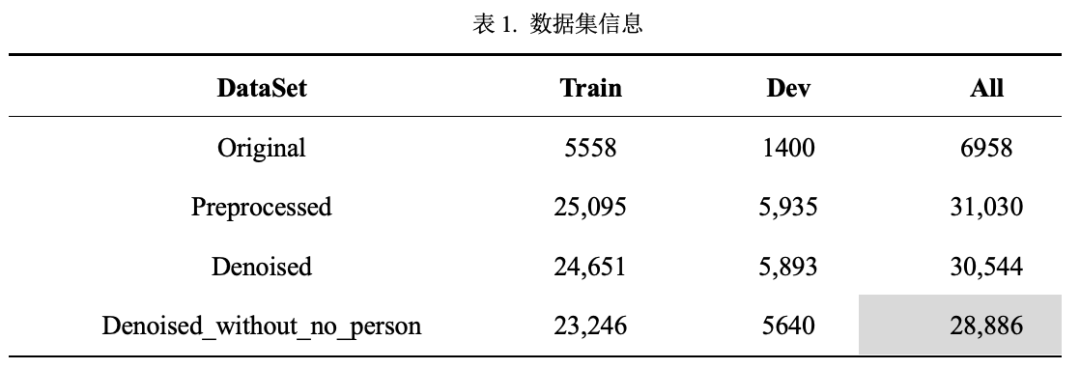• Original：官方提供的原始数据集train.txt。

• Preprocessed：将Original数据重新整理，将“被告人集合”拆分成单独的“被告人”。

• Denoised：去除Preprocessed中，同时满足问题（1）和（2）的样本。

• Denoised_without_no_person：去除Denoised中，存在问题（1）的样本。

def pad_data(file_name, tokenizer, max_len):
"""
This function is used as the Dataset Class in PyTorch
"""
# configuration:
file_content = json.load(open(file_name, encoding='utf-8'))
data = []

for line in file_content:
paragraph = line['paragraph']
person = line['person']
element = line['element_name']
sentence = line['sentence']
ovalue = line["ovalue"]
label = line['label']

sentence_a = add_dollar2person(person) + element
sentence_b = add_star2sentence(sentence, ovalue)

src_id, sent_id = tokenizer.encode(sentence_a, sentence_b, truncate_to=max_len-3)      # 3 special tokens

# pad src_id and sent_id (with 0 and 1 respectively)
src_id = np.pad(src_id, [0, max_len-len(src_id)], 'constant', constant_values=0)
sent_id = np.pad(sent_id, [0, max_len-len(sent_id)], 'constant', constant_values=1)

data.append((src_id, sent_id, label))
return data

def make_batches(data, batch_size, shuffle=True):
"""
This function is used as the DataLoader Class in PyTorch
"""
if shuffle:
np.random.shuffle(data)
for j in range(len(data)//batch_size):
one_batch_data = data[j * batch_size:(j + 1) * batch_size]
src_id, sent_id, label = zip(*one_batch_data)

src_id = np.stack(src_id)
sent_id = np.stack(sent_id)
label = np.stack(label).astype(np.float32)  # change the data type to compute BCELoss conveniently



def train(model, dataset, lr=1e-5, batch_size=1, epochs=10):

max_steps = epochs * (len(dataset) // batch_size)
# max_train_steps = args.epoch * num_train_examples // args.batch_size  // dev_count
# learning_rate=LinearDecay(lr, int(0), max_steps),
learning_rate=lr,
parameter_list=model.parameters(),
weight_decay=0)

model.train()
logging.info('start training process!')
for epoch in range(epochs):
# shuffle the dataset every epoch by reloading it
data_loader = make_batches(dataset, batch_size=batch_size, shuffle=True)

running_loss = 0.0
for i, data in enumerate(data_loader):
# prepare inputs for the model
src_ids, sent_ids, labels = data

# convert numpy variables to paddle variables
src_ids = D.to_variable(src_ids)
sent_ids = D.to_variable(sent_ids)
labels = D.to_variable(labels)

# feed into the model
outs = model(src_ids, sent_ids, labels=labels)

loss = outs
loss.backward()
optimizer.minimize(loss)

running_loss += loss.numpy()
if i % 10 == 9:
print('epoch: ', epoch + 1, '\tstep: ', i + 1, '\trunning_loss: ', running_loss)
running_loss = 0.0

state_dict = model.state_dict()
F.save_dygraph(state_dict, './saved/plan3_all/model_'+str(epoch+1)+'epoch')
print('model_'+str(epoch+1)+'epoch saved')

logging.info('all model parameters saved!')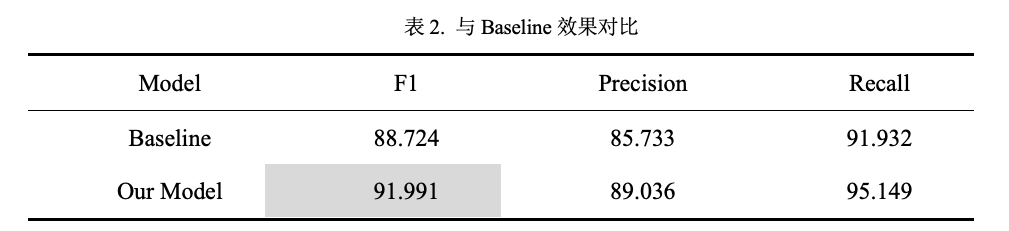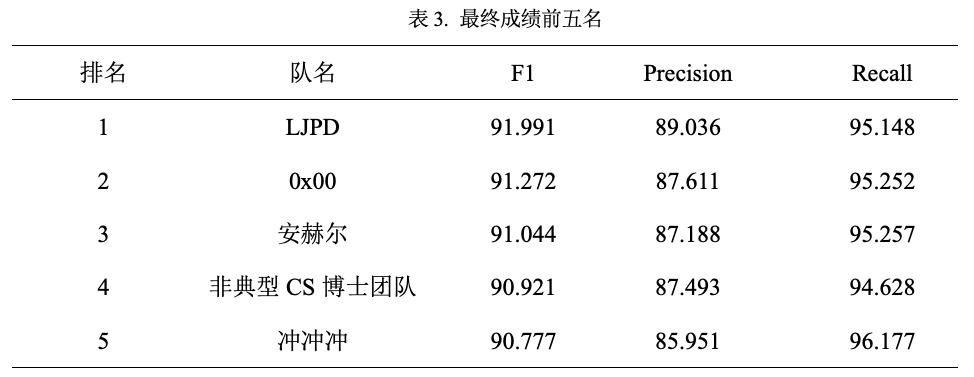（1） 向前扩句后，句子中仍不包含被告人的情况；

（2） 输入序列较长的情况（分词之后达到1000个token以上）

（1） 滑窗策略：若句子中不包含被告人，则使用该句之前的所有信息（或者直接输入段落）。这样输入序列的长度会大幅增加，这时采用多个ERNIE 512窗口，stride=128，对完整序列进行滑窗，不同窗口重叠的地方采用pooling的方式获取最终隐藏状态。这样就打破了ERNIE输入512长度的限制；

（2） 拼接关键向量：在滑窗策略中，输入序列增加之后，相应的冗余信息也会增加。因此我们将进一步对【被告人】和【要素原始值】的信息进行增强。现有的方案是使用[CLS]位置的最终隐藏层向量连接全连接层进行二分类，我们可以将【被告人】和【要素原始值】每个token位置的最终隐层向量进行取平均，然后和[CLS]位置的向量进行拼接，将原先768维的向量扩展到2304维，使用新的向量进行二分类。

下载安装命令

## CPU版本安装命令

## GPU版本安装命令### 作者的其它热门文章

0
0 收藏

0 评论
0 收藏
0